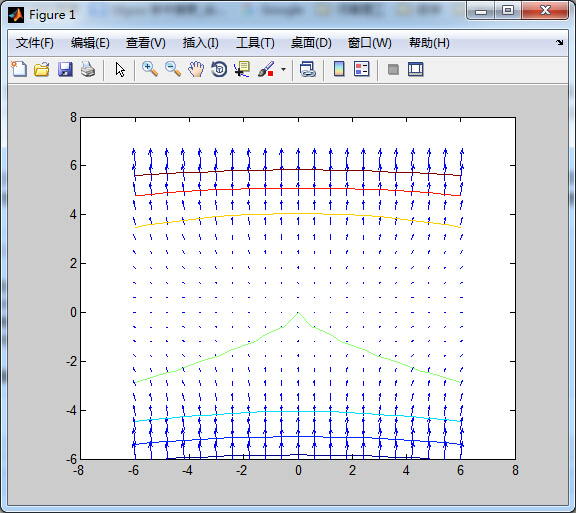• matlabfind函数的使用说明——emily语法介绍
2021-04-18 06:49:38

find

找到非零元素的索引和值

语法：

1. ind = find(X)

2. ind = find(X, k)

3. ind = find(X, k, 'first')

4. ind = find(X, k, 'last')

5. [row,col] = find(X, ...)

6. [row,col,v] = find(X, ...)

说明：

1. ind = find(X)

找出矩阵X中的所有非零元素，并将这些元素的线性索引值(linear indices：按列)返回到向量ind中。

如果X是一个行向量，则ind是一个行向量；否则，ind是一个列向量。

如果X不含非零元素或是一个空矩阵，则ind是一个空矩阵。

2. ind = find(X, k) 或

3. ind = find(X, k, 'first')

返回第一个非零元素k的索引值。

k必须是一个正数，但是它可以是任何数字数值类型。

4. ind = find(X, k, 'last')

返回最后一个非零元素k的索引值。

5. [row,col] = find(X, ...)

返回矩阵X中非零元素的行和列的索引值。

这个语法对于处理稀疏矩阵尤其有用。

如果X是一个N(N>2)维矩阵，col包括列的线性索引。

例如，一个5*7*3的矩阵X，有一个非零元素X(4,2,3)，find函数将返回row=4和col=16。也就是说，(第1页有7列)+(第2页有7列)+(第3页有2列)=16。

更多相关内容
• 您想要选择一个元素if>非零的行cumsum是1 AND>非零的列cumsum是1 AND>元素本身不为零.以下代码解决了这个问题：A = [0, 0, 3, 4;4, 3, 2, 0;2, 0, 2, 0];batches = cell(0);while any(A(:)~=0)selector = ...

冈瑟已经走上了正轨.您想要选择一个元素if

>非零的行cumsum是1 AND

>非零的列cumsum是1 AND

>元素本身不为零.

以下代码解决了这个问题：

A = [0, 0, 3, 4;

4, 3, 2, 0;

2, 0, 2, 0];

batches = cell(0);

while any(A(:)~=0)

selector = cumsum(A~=0, 1) .* cumsum(A~=0, 2) .* (A~=0) == 1;

batches{end+1} = A .* selector;

A(selector) = 0;

end

但请注意,返回的解决方案并非最佳,因为它的第二批是

0 0 0 4

0 3 0 0

2 0 0 0

这意味着剩余的矩阵元素来自同一列：

0 0 0 0

0 0 2 0

0 0 2 0

不幸的是,你无法在同一批次中绘制它们.所以你最终只有四批而不是三批.

编辑：可能首先选择那些出现在具有大量非零的行/列中的元素是个好主意.例如,可以使用这些权重

weight = repmat(sum(A~=0, 1), size(A, 1), 1) ...

.* repmat(sum(A~=0, 2), 1, size(A, 2)) .* (A~=0)

weight =

0 0 6 2

6 3 9 0

4 0 6 0

以下算法

batches = cell(0);

while any(A(:)~=0)

batch = zeros(size(A));

weight = repmat(sum(A~=0, 1), size(A, 1), 1) ...

.* repmat(sum(A~=0, 2), 1, size(A, 2)) .* (A~=0);

while any(weight(:)~=0)

[r,c] = find(weight == max(weight(:)), 1);

batch(r,c) = A(r,c);

A(r,c) = 0;

weight(r,:) = 0;

weight(:,c) = 0;

end

batches{end+1} = batch;

end

返回那些批次.

batches{:}

ans =

0 0 0 4

0 0 2 0

2 0 0 0

ans =

0 0 3 0

4 0 0 0

0 0 0 0

ans =

0 0 0 0

0 3 0 0

0 0 2 0

所以它至少适用于这个小测试用例.

展开全文• 此函数采用大小向量 [mn] 和连接标量（默认为 4，或 8），并返回一个 p×2 数组，其中包含 m×n 数组中所有相邻元素对的线性索引。 请注意，p 不等于 (m*n*conn)，因为边框元素没有内部元素那么多的邻居。 我创建此...
• matlab 如何把一个矩阵中的非零数取出组成一行>... B=nonzeros(A)' B = 4 2 2 3 4 3 1 2 3 2matlab中怎么求数组中非零元素的个数matlab中求矩阵中非零元素的均值怎么求啊？ 代码： a(a==0)=NaN; mean(...

matlab 如何把一个矩阵中的非零数取出组成一行

>> A=randi([0 4],4) A = 4 2 1 0 2 3 2 3 0 4 0 2 0 3 0 0 >> B=nonzeros(A)' B = 4 2 2 3 4 3 1 2 3 2

matlab中怎么求数组中非零元素的个数matlab中求矩阵中非零元素的均值怎么求啊？ 代码： a(a==0)=NaN; mean(a,'omitnan') 把零替换成NaN，然后再用mean函数的'omitnan'参数计算均值，相比其他方法可以避免find函数或者判别式占用矩阵索引参数。比如一个多维矩阵A(x,y,z,)某一维的非零值计算均值，可用如上方法。

matlab中如何把一个矩阵中非零元素都变为1？

在Matlab中，空矩阵、零矩阵和全一矩阵是比较特殊的矩阵，它们在结构形式和矩阵元素的组织上有着独特的规律性。matlab中如何把一个矩阵中非零元素都变为1的方法是：

利用ones(N)生成一个N

Matlab如何提取非零元素

输入a=(x~=0)进行比较各个元素是否为0，如下图所示。

按回车键之后，可以看到x数组各个元素是否为0，为0就显示为0，不是就显示为1，如下图所示。

>> A = [-1 0 2;-3 -4 0;9 -78 1] A = -1 0 2 -3 -4 0 9 -78 1 >> A(A~=0) = 1 A = 1 0 1 1 1 0 1 1 1

MATLAB 中我想提取一列数据中所有非零的数并组成新比如一列数据[0,0,0,1,75,13,12,0,0,124,0,0,1216,87,3,6,3,0,0]; 想要假设原来的矩阵叫做A a=size(A,2); for i=1:a if A(i)==0 A(i)==[]; i=i-1; b=size(A,2); if i==b break end end end A 我猜的。

矩阵非空数据个数，matlab怎么统计？

矩阵NaN元素可用以下式子求得： num=numel(find(isnan(a)))； 因此非空数据个数： numel(a)-numel(find(isnan(a))) >> a a = 1 6 2 7 3 NaN 4 NaN 5 10 >> numel(a)-numel(find(isnan(a))) ans = 8 >>

小白求解： matlab 找出矩阵中的非零列

比如我现在有一个2*9的矩阵，A=, B=。现在我A=; B=; index = A&B; A(index) B(index) 望喜欢

在matlab里如何获得一个矩阵的行数或列数

在matlab里可以利用size函数获得一个矩阵的行数或列数。 size(a) 返回一个行向量，其元素包含 A 的相应维度的长度。例如，如果 a 是一个2×3矩阵，则 size(a) 返回向量 [2,3]。 具体说明如下。

第一步在matlab中命令行窗口中输入“a=[1 2 3;2 4

展开全文• matlab函数学习，unique, digits & vpa, DCTMTX，以及自定义函数。

参考链接：
Matlab中保持原来数值顺序的unique函数实现https://raocong.blog.csdn.net/article/details/6861576

function uniVecUnsorted = unique_unsorted(vector)

digits(5);	%控制数据精度
uniValues = vpa(unique(vector));	%注意vpa的位置，放在其他地方会导致uniValues的值按科学计数法显示
mask = zeros( size(vector, 1), size(vector, 2));

for i = 1 : length(uniValues(i));
maskValue = (vector == uniValues(i));		%将值相同元素的位置上的flag置为1
indFirst = find(vector == uniValues(i), 1, 'first');	%找到第一个值相同元素所在的位置
maskValue(indFirst + 1 : end) = 0;		%将其他值相同元素的位置上的flag值置为0

end

end

function output = IntDCT8

digits(5);
origin_DCT = vpa(DCTMTX(8))
b = vpa (unique_unsorted(abs(origin_DCT))	%注意vpa的位置，放在其他地方会导致uniValues的值按科学计数法显示

end


调用方法：
在command window输入“IntDCT8”.

输出结果：

b =
.35355
.49039
.41573
.27779
.97545e-1
.46194
.19134

展开全文• Matlab矩阵元素与子矩阵的提取>> %矩阵元素的提取>> A=[1 2 3;4 5 6;7 8 9;10 11 12]A =1 2 34 5 67 8 910 11 12&... %通过元素序号提取元素(矩阵元素序号与它的存储顺序是一一对应的！)>> A(6)a...
• 从数组中提取子数组很简单： a = 1:10; a(4:8) % 返回元素 4-8。 但有时，我有一个返回数组的函数，但我只想要该数组的一个子集： a = foo(x,y,z); b = a(2,9:end); 这里的 'a' 是一个临时变量，会污染我的工作...
• 最近在做毕设时遇到需要将数据存放到三维数组中进行保存的问题，然鹅~保存数据容易提取难，具体说来是提取数据的索引上遇到了问题，但经过我不懈努力，终于搞懂了，算是在find函数上的小小进阶~hhh好开心~~~
• OpenCV和matlab都提供了强大的矩阵处理功能。但matlab也有一些功能是openCV不具备的。如find函数，可以找出矩阵中某个特定值的行列号。本文介绍一种方法，可以模拟find函数的功能。// OpenCVFind.cpp : 定义控制台...
• matlab中删除相邻相同元素关注:126答案:2手机版解决时间 2021-03-06 01:51提问者你說、你愛我2021-03-05 20:49A=[zx as cd cd re tf cd df df df gh]如何删除相邻的相同数据变成B=[zx as cd re tf cd df gh]谢谢指教...
• 1 3 >> x=[1,0,5,8] x = 1 0 5 8 >> y=find(x) y = 1 3 4 >> >> y1=2 y1 = 2 >> y2=9 y2 = 9 >> y3=-1 y3 = -1 >> if (y1>z) z=y1 elseif (y2>z) z=y2 else z=y3 end z = 2 >> 1.find函数用来寻找非0元素的...
• find函数语法如下,我们着重讨论下返回值的问题,并对二维以上数组进行查找 1.k = find(X) 2.k = find(X,n) 3.k = find(X,n,direction) 4.[row,col] = find(___) 5.[row,col,v] = find(___)     一、前三个语法...三维数组
• 使用bsxfun的冒号模拟功能,利用两个bsxfun(@le和bsxfun(@ge – )N = size(A,1)[R,~] = find((bsxfun(@le,B(:,1),1:N) & bsxfun(@ge,B(:,2),1:N)).')out = A(R,:)样品运行#1 –>> AA =1 2 34 5 67 8 ...
• 我必须使用2D CT图像进行一个项目,并使用Matlab(仅)对肝脏和肿瘤进行分割.最初我必须单独分割肝脏区域.我使用区域生长进行肝脏分割.它获得种子点作为输入.输出是具有肝区边界的图像.现在我需要仅由边界包围的区域.我...
• 希望它能带给你创意……我所做的基本上是中值滤波器过滤图像(就像你做的那样)并使用bwareaopen删除小元素.然后我打电话给regionprops来获得一堆属性,其中最重要的是区域和偏心.这个想法是所有字母“a”应该有一个...
• 诚教:matlab中取矩阵的其中几行几列如果想取一个矩阵其中的第1,3,5,6,8行,为一个新的矩阵,则下列语句：B=A([1,3,5,6,8],:);如果是取第1,3,5行和第1,4,5列相交的数据为新矩阵,可以表示为：C=A([1,3matlab中矩阵的...
• 解决方案 There is a function to find the number of nonzero matrix elements nnz. You can use this function on a logical matrix, which will return the number of true. In this case, we apply nnz on the ...
• matlab怎么把矩阵非零元素个数赋值给一个变量1.find函数tql一、find函数是什么？二、使用步骤1.语法说明Syntax2.Description3. length(find([1 4 10 1 0 0]))=44. size(find([1 4 10 1 0 0]), 2)=4总结 1.find函数...算法
• 1.求矩陣中各個不同的元素出現的次數tabulateFrequency table.TABLE = tabulate(X) takes a vector X and returns a matrix, TABLE.The first column of TABLE contains the unique values of X. Thesecond is the ...
• 用Matlab实现碳纤维边缘的提取并输出坐标 一、 图像处理算法流程 1. 读取图片： image = imread(‘1.jpg’)，在Matlab中，常用于读取图片的函数为imread()。 2. 转换为灰度图 读取的图片可能为彩色图片，也有可能...
• 给定一个数组,如何让matlab生成一个这个数组中的一个随机数?x=;x(randi(length(x)));其中randi(length(x))生成从1~(x的长度)这几个自然数中随即的一个数让这个数作为下标,就可以从x中随即选取一个数x...
• 如果cell中每个元素是字符串, ismember 慢于 strcmp a_cell = {'ni', 'hao'} % 第一种方法 idx = find(ismember(a_cell, 'ni' )) % 第二种方法 idx = find(stcmp(a_cell, 'ni' )) 其中 第二种方法更快 ...
• 下面是从文件中提取需要的数据的MATLAB程序。clcclear allfnm = 'b.duff2';%文件名fid = fopen(fnm,'r');%打开文件dt = {};%存储文件内容。n = 1;%文件数据行号while ~feof(fid) %判断是否到...
• 查找某个元素在向量中的位置：m = find(A==5); %A是向量，5是要查找的元素值，返回位置m 查找某个元素在矩阵中的位置：[m,n] =find(B==5); %B是矩阵，5是要查找的元素值，返回行位置m和列位置n 2. 字符型/cell型 ...
• Matlab——常用函数的用法总结(部分直接摘自mathwork，持续更新)一、绘图篇1.图象显示形式①figure(创建图窗窗口)figure：使用默认属性值创建一个新的图窗窗口。生成的图窗为当前图窗(当前图窗就是你下一次的画图...
• 基于MATLAB的图片中文字的提取及识别一．引言随着计算机科学的飞速发展，以图像为主的多媒体信息迅速成为重要的信息传递媒介，在图像中，文字信息(如新闻标题等字幕) 包含了丰富的高层语义信息，提取出这些文字，...python opencv 计算机视觉 机器学习
•边缘检测 opencv 计算机视觉 算法
• 1. Matlab中数组元素引用有三种方法：下标法(subscripts)索引法(index)布尔法(Boolean)注意：在使用这三种方法之前，大家头脑一定要清晰的记住，Matlab中数组元素是按列存储(与Fortran一样)，比如说下面的二维数组：......

# matlab用find提取负元素matlab 订阅